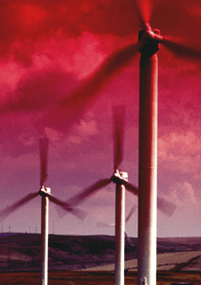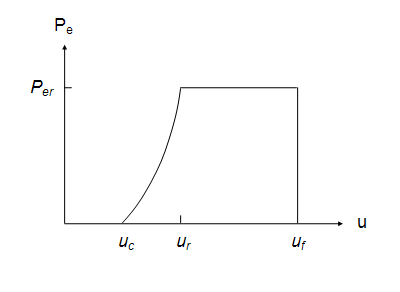Evaluating the Average Power Delivered by a Wind Turbine

This example uses Symbolic Math Toolbox and the Statistics and Machine Learning Toolbox to explore and derive a parametric analytical expression for the average power generated by a wind turbine.The parametric equation can be used for evaluating various wind turbine configurations and wind farm sites. More information see Wind Resource Assessment.

Background

The total power delivered to a wind turbine can be estimated by taking the derivative of the wind's kinetic energy. This results in the following expression:

${\mathit{P}}_{\mathit{w}}=\frac{\rho {\text{\hspace{0.17em}}\mathit{A}\text{\hspace{0.17em}}\mathit{u}}^{3}}{2}$ (1)

• A is the swept area of turbine blades, in ${\mathit{m}}^{2}$

• ρ = air density, in $\mathrm{kg}/{m}^{3}$

• u = wind speed, in $\mathit{m}/\mathit{s}$

The process of converting wind power to electrical power results in efficiency losses, as described in the diagram below.The electrical power output of a practical wind turbine can be described using the following equation:

${\mathit{P}}_{\mathit{e}}=\frac{{\mathit{C}}_{\mathrm{tot}}\text{\hspace{0.17em}\hspace{0.17em}}\rho {\text{\hspace{0.17em}}\mathrm{Au}}^{3}}{2}$ (2) where ${\mathit{C}}_{\mathrm{tot}}=\mathrm{overall}\text{\hspace{0.17em}}\mathrm{efficiency}={\mathit{C}}_{\mathit{p}}{\mathit{C}}_{\mathit{t}}{\mathit{C}}_{\mathit{g}}$

The overall efficiency is between 0.3 and 0.5, and varies with both wind speed and rotational speed of the turbine. For a fixed rotational speed, there is a rated wind speed at which electrical power generated by the wind turbine is near its maximum (${\mathit{P}}_{\mathrm{er}}$), and overall efficiency at this point is denoted ${\mathit{C}}_{\mathrm{totR}}$.

${\mathit{P}}_{\mathrm{er}}=\frac{{\mathit{C}}_{\mathrm{totR}}\text{\hspace{0.17em}\hspace{0.17em}}\rho {\text{\hspace{0.17em}}\mathrm{Au}}^{3}}{2}$ (3)

Assuming a fixed rotational speed, the electrical power output of the wind turbine can be estimated using the following profile:Where

• ${\mathit{u}}_{\mathit{r}}$ = rated wind speed

• ${\mathit{u}}_{\mathit{c}}$ = cut-in speed, the speed at which the electrical power output rises above zero and power production starts

• ${\mathit{u}}_{\mathit{f}}$ = furling wind speed, the speed at which the turbine is shut down to prevent structural damage

As seen in the figure, we assume that output power increases between ${u}_{c}$ and ${u}_{r}$, then is at a constant maximum value between ${\mathit{u}}_{\mathit{r}}$ and ${\mathit{u}}_{\mathit{f}}$. Power output is zero for all other conditions.

I. Define piecewise expression for power

We define a piecewise function that described turbine power.

syms Per C_1 C_2 k u u_c u_f u_r
Pe = piecewise(u < u_c, 0, u_c <= u <= u_r, C_1 + C_2 * u^k, (u_r <= u) <= u_f, Per, u_f < u, 0)
Pe =

Where variables ${\mathit{C}}_{1}$ and ${\mathit{C}}_{2}$ are defined as follows:

C_1 = (Per * u_c^k)/(u_c^k - u_r^k)
C_1 =

$\frac{\mathrm{Per} {{u}_{c}}^{k}}{{{u}_{c}}^{k}-{{u}_{r}}^{k}}$

C_2 = Per/(u_r^k - u_c^k)
C_2 =

$-\frac{\mathrm{Per}}{{{u}_{c}}^{k}-{{u}_{r}}^{k}}$

II. Define external wind conditions

The rated power output offers a good indication of a how much power a wind turbine is capable of producing, however we'd like to estimate how much power (on average) the wind turbine will actually deliver. To calculate average power, we need to account for external wind conditions. A Weibull distribution does a good job at modeling the variance in wind, therefore the wind profile can be estimated using the following probability density function:

$f\phantom{\rule{-0.16666666666666666em}{0ex}}\left(u\right)=\frac{\left(\frac{b}{a}\right)\phantom{\rule{0.16666666666666666em}{0ex}}{\left(\frac{u}{a}\right)}^{b-1}}{{\mathrm{e}}^{{\left(\frac{u}{a}\right)}^{b}}}$ (4)

In general, larger 'a' values indicate a higher median wind speed, and larger 'b' values indicate reduced variability.

We use the Statistics and Machine Learning Toolbox to generate a Weibull distribution and illustrate the variability in wind at our wind farm site (a=12.5, b=2.2):

a = 12.5;
b = 2.2;
N = 1000;
pd = makedist('Weibull','a',a,'b',b)
pd =
WeibullDistribution

Weibull distribution
A = 12.5
B =  2.2

r = wblrnd(a,b,[1 N])
r = 1×1000

6.0811    4.3679   17.3751    4.1966    8.7677   18.3517   13.9761    9.9363    3.0039    2.7496   16.5233    2.5333    3.0151   10.7854    6.3169   16.9442   11.6922    4.1418    6.4460    2.9379    8.4449   21.6033    5.4887    3.6903    8.1241    6.9789    7.1974   12.1293    8.4485   16.1833    7.7371   21.9390   14.0043   20.8297   18.3668    5.9351    7.8970   13.3122    3.2335   21.7093   11.4461   12.2905    6.8609    6.3983   15.8128   10.7241   11.3478    8.5754    7.6896    7.0249

x = linspace(0,34,N);
y = pdf(pd,x);
plot(x,y,'LineWidth',2)
hold on
histogram(r,15,'Normalization','pdf')
hold off
title('Weibull Distribution of Wind Speeds')
xlabel('Wind Speed (m/s)')III. Calculate average power

The average power output from a wind turbine can be obtained using the following integral:

$P{e}_{average}={\int }_{0}^{\infty }Pe\left(u\right)\phantom{\rule{0.16666666666666666em}{0ex}}f\left(u\right)du$ (5)

Power is zero when wind speed is less than the cut in wind speed ${u}_{c}$ and greater than furling wind speed ${\mathit{u}}_{\mathit{f}}$. Therefore, the integral can be expressed as follows:

$P{e}_{average}={C}_{1}\phantom{\rule{0.16666666666666666em}{0ex}}\left({\int }_{{u}_{c}}^{{u}_{r}}f\phantom{\rule{-0.16666666666666666em}{0ex}}\left(u\right)\phantom{\rule{0.16666666666666666em}{0ex}}du\right)+{C}_{2}\phantom{\rule{0.16666666666666666em}{0ex}}\left({\int }_{{u}_{c}}^{{u}_{r}}{u}^{b}f\phantom{\rule{-0.16666666666666666em}{0ex}}\left(u\right)du\right)+\mathrm{P}\mathrm{e}\mathrm{r}\phantom{\rule{0.16666666666666666em}{0ex}}\left({\int }_{{u}_{r}}^{{u}_{f}}f\phantom{\rule{-0.16666666666666666em}{0ex}}\left(u\right)\phantom{\rule{0.16666666666666666em}{0ex}}du\right)$ (6)

There are two distinct integrals in equation (7). We plug equation (4) into these integrals and simplify them using the substitutions: $x={\left(\frac{u}{a}\right)}^{b}$ and $\mathrm{d}\mathrm{x}=\left(\frac{b}{a}\right)\phantom{\rule{0.16666666666666666em}{0ex}}{\left(\frac{u}{a}\right)}^{b-1}\phantom{\rule{0.16666666666666666em}{0ex}}\mathrm{d}\mathrm{u}$. This simplifies our original integrals to the following:

$\int f\phantom{\rule{-0.16666666666666666em}{0ex}}\left(u\right)\phantom{\rule{0.16666666666666666em}{0ex}}du=\int \frac{1}{{\mathrm{e}}^{x}}\phantom{\rule{0.16666666666666666em}{0ex}}dx$ (7)

$\int {u}^{b}\phantom{\rule{0.16666666666666666em}{0ex}}f\phantom{\rule{-0.16666666666666666em}{0ex}}\left(u\right)\phantom{\rule{0.16666666666666666em}{0ex}}du={a}^{b}\phantom{\rule{0.16666666666666666em}{0ex}}\left(\int \frac{x}{{\mathrm{e}}^{x}}\phantom{\rule{0.16666666666666666em}{0ex}}dx\right)$ (8)

Solving these integrals and then replacing x with ${\left(\frac{u}{a}\right)}^{b}$ yields:

syms a b x
int1 = int(exp(-x), x);
int1 = subs(int1, x, (u/a)^b)
int1 =

$-{\mathrm{e}}^{-{\left(\frac{u}{a}\right)}^{b}}$

int2 = int(x * exp(-x) * a^b, x);
int2 = subs(int2, x, (u/a)^b)
int2 =

$-{a}^{b} {\mathrm{e}}^{-{\left(\frac{u}{a}\right)}^{b}} \left({\left(\frac{u}{a}\right)}^{b}+1\right)$

Substituting the results into equation (6) yields an equation for average power output of the wind turbine.

Peavg = subs(C_1 * int1, u, u_r) - subs(C_1 * int1, u, u_c) + subs(C_2 * int2, u, u_r) - subs(C_2 * int2, u, u_c) + subs(Per * int1, u, u_f) - subs(Per * int1, u, u_r)
Peavg =

IV. Conclusion

We have used the Symbolic Math Toolbox to develop a parametric equation which can be used to perform simulation studies to determine the average power generated for various wind turbine configurations and wind farm sites.

Symbolic Math Toolbox Documentation

Mathematical Modeling with Symbolic Math Toolbox

Get examples and videos